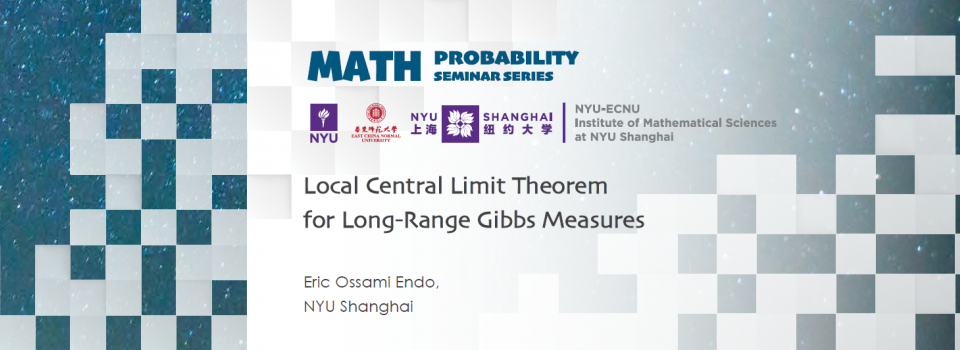# Local Central Limit Theorem for Long-Range Gibbs MeasuresTopic:
Local Central Limit Theorem for Long-Range Gibbs Measures
Date & Time:
Thursday, October 20, 2022 - 17:00 to 18:00
Speaker:
Eric Ossami Endo, NYU Shanghai
Location:

- RSVP Here -

Abstract:

The Local Central Limit Theorem is a result that provides a more refined result than the Central Limit Theorem. For the classic lattice case, it studies the convergence of the probability of the events S_k=n for an integer n, where S_k is a properly scaled sum of i.i.d. random variables.

Both Central Limit Theorem and Local Central Limit Theorem were vastly studied for random variables generated by models coming from Statistical Mechanics. Several papers such as [1,2,3] proved that, under certain conditions for the potentials, assuming that the Central Limit Theorem holds for random fields defined on the lattice, then the Local Central Limit Theorem will hold as well.

Considering a sufficiently high-temperature regime, we prove in  that for a sequence of Gibbs measures with two-body long-range, absolutely summable potentials on the lattice with spins taking lattice distributed values, for which the Integral Central Limit Theorem is satisfied, then the Local Central Limit Theorem also holds for that sequence. Our result complements , where some families of absolutely summable long-range potentials that fail the condition in  still satisfy the result at sufficiently high temperatures.

References

 M. Campanino, D. Capocaccia, and B. Tirozzi. The local central limit theorem for a Gibbs random field. Comm. Math. Phys. 70(2): 125–132, 1979.
 G. Del Grosso. On the local central limit theorem for Gibbs processes. Comm. Math. Phys. 37(2): 141–160, 1974.
 R.L. Dobrushin, and B. Tirozzi. The central limit theorem and the problem of equivalence of ensembles. Comm. Math. Phys. 54(2): 173–192, 1977.
 E.O. Endo, and V. Margarint. Local Central Limit Theorem for Long-Range Two-Body Potentials at Sufficiently High Temperatures. Journal of Statistical Physics. 189(34), 2022.

Biography:

Endo obtained a double Ph.D. degree in Applied Mathematics at the University of São Paulo, in Brazil, under the supervision of Professor R. Bissacot (2018), and in Mathematics at the University of Groningen, in the Netherlands, under the supervision of Professor A.C.D. van Enter and Professor D. Valesin (2018). Endo’s Ph.D. thesis received the Cum Laude distinction at the University of Groningen. He started his career as a Postdoctoral Instructor at NYU Shanghai under the supervision of Professor R. Fernández (2018-2021). Currently, he is an Assistant Professor of Practice in Mathematics at NYU Shanghai. His interests are statistical mechanics of ferromagnetic models, thermodynamic formalism, and combinatorics.

Seminar by the NYU-ECNU Institute of Mathematical Sciences at NYU Shanghai